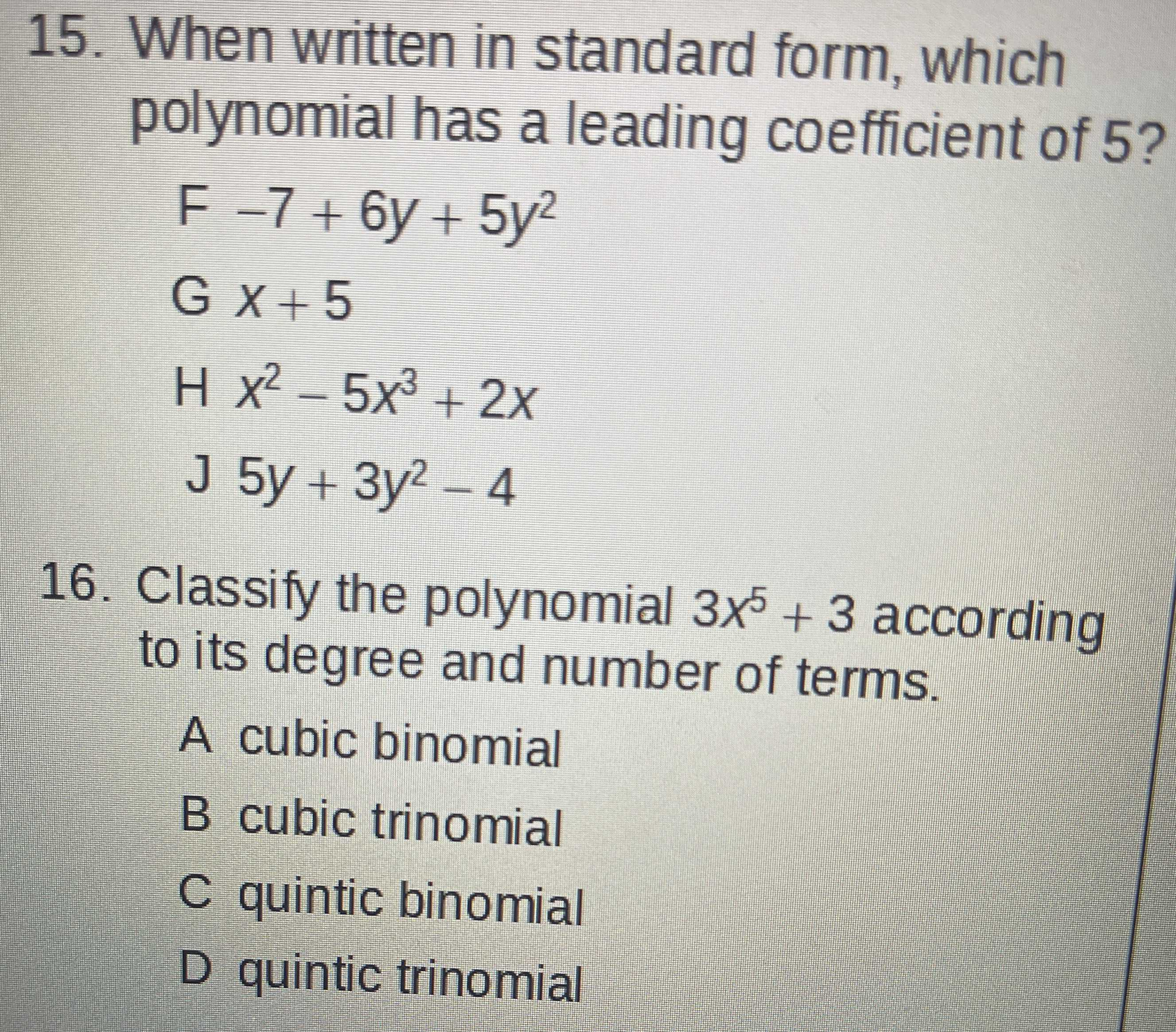### ¿Todavía tienes preguntas de matemáticas?

Pregunte a nuestros tutores expertos
Algebra
Pregunta15. When written in standard form, which polynomial has a leading coefficient of $$5$$ ?

$$- 7 + 6 y + 5 y ^ { 2 }$$

$$x + 5$$

$$x ^ { 2 } - 5 x ^ { 3 } + 2 x$$

$$5 y + 3 y ^ { 2 } - 4$$

16. Classify the polynomial $$3 x ^ { 5 } + 3$$ according to its degree and number of terms.

A cubic binomial

B cubic trinomial

C quintic binomial

D quintic trinomial